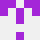# Lua比较两个表的值（不考虑键的顺序）

``````function TableCompareNoOrder(table1,table2)
if #table1 ~= #table2 then return false end
local equal = false
for key, item in pairs(table1) do
for key2, item2 in pairs(table2) do
if item == item2 then equal = true end
end
if equal == false then return false end
end
local equal = false
for key, item in pairs(table2) do
for key2, item2 in pairs(table1) do
if item == item2 then equal = true end
end
if equal == false then return false end
end

return equal
end

a = {1,2,3,0}
b = {2,3,1,0}
print(TableCompareNoOrder(a,b))
``````stackoverflow用户7185318

``````function TableCompareNoOrder(table1, table2)
if #table1 ~= #table2 then return false end
-- 如果table1 == table2，则在此处考虑早期的"return true"
local t1_counts = {}
-- 检查相同的元素出现相同的次数
for _, v1 in ipairs(table1) do
t1_counts[v1] = (t1_counts[v1] or 0) + 1
end
for _, v2 in ipairs(table2) do
local count = t1_counts[v2] or 0
if count == 0 then return false end
t1_counts[v2] = count - 1
end
return true
end
``````

``````function TableCompareNoOrder(table1, table2)
if #table1 ~= #table2 then return false end
-- 懒惰的实现: 对两个表的副本进行排序，而不是使用二分查找。需要两倍的内存。
local t1_sorted = {table.unpack(table1)} --简单复制表的方法，受堆栈大小限制
table.sort(t1_sorted)
local t2_sorted = {table.unpack(table2)}
table.sort(t2_sorted)
for i, v1 in ipairs(t1_sorted) do
if t2_sorted[i] ~= v1 then return false end
end
return true
end
``````

2022-03-05 13:13:15